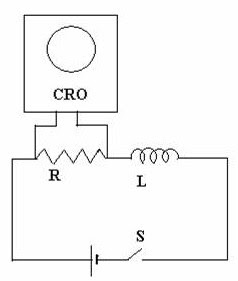## Pages

`“Life is like riding a bicycle.  To keep your balance you must keep moving.”–Albert Einstein`

## Tuesday, April 12, 2011

### AP Physics C – Additional Practice Questions (MCQ) on Electromagnetic Induction

"There's a way to do it better - find it"
Thomas A. Edison

In my last post I had given you a few multiple choice practice questions (with solution)
involving electromagnetic induction. Today we will discuss a few more questions in this section. These are meant for AP Physics C aspirants even though AP Physics B aspirants also will find them useful:

(1) The adjoining figure shows a 1000 turn coil of fine insulated copper wire connected to the y-inputs A and B of a cathode ray oscilloscope set for displaying the voltage wave form induced in the coil. A bar magnet, with its axis vertical and coinciding with the axis of the coil, is initially at rest, with its centre O at a height 10 cm from the centre of the coil. The bar magnet is allowed to fall freely under gravity and the induced voltage as a function of time is displayed on the screen of the oscilloscope. Which one among the following graphs best represents the induced volta

The magnetic flux linked with the coil increases up to the instant when the centres of the magnet and the coil coincide. Thereafter the magnetic flux decreases. Therefore, the direction of the induced emf gets reversed. The reversal of the induced emf occurs at the instant when the centres of the magnet and the coil coincide since the rate of change of magnetic flux at that instant is zero even though the flux linkage is maximum. Further, the latter half of the induced voltage has a peak of greater magnitude since the speed of fall of the magnet is greater so that the rate of change of magnetic flux is greater. The correct option is (a).(2) The voltage variation across a resistance R in a series LR circuit is displayed as a function of time using a cathode ray oscilloscope. The swith S is closed at time t = 0 and then opened at time t = t1. Which one among the following graphs best represents the voltage variation across R during the time interval 0 to t1?

When the switch S is closed,the time constant of the circuit is L/R and is significant. The current I in the circuit therefore does not rise abruptly to the final maximum value I0 (let us say). . The rise of current is exponential and hence the voltage across the resistance R rises exponentially with time.

[The exponential growh of the current I is given by I = I0(1 – eRt/L) where e is the base of natural logarithms].

When the switch S is opened at the instant t1 the open circuit at the switch makes the resistance of the circuit infinite and hence the time constant becomes zero. The current drops abruptly to zero. The voltage drop across R also drops abruptly to zero. The correct option is (e).

[The graph (d) may distract you. The growth of the voltage shown in it is not exponential. In an exponential growth, the initial rate of growth will be the largest].

(3) The switch S in the circuit shown is closed and sufficient time is allowed so that the current through the inductance L and the resistance R becomes the final steady value. The inductance L is about 20 henry and the resistance R is about 10 ohm. The switch S is opened at time t = 0. Then the voltmeters V1 and V2 will indicate the same reading at

(a) time t = 0

(b) time t = L/R

(c) rime t = 2 L/R

(d) time t = L/2R

(e) all times

This question is very simple but it may confuse you.

Since the LR circuit has a non-zero time constant (L/R), the voltmeter readings will not become zero abruptly but will drop to zero exponentially with time. Since the inductance and resistance are connected in parallel, the voltmeters V1 and V2 will indicate the same reading at all times [Option (e)].